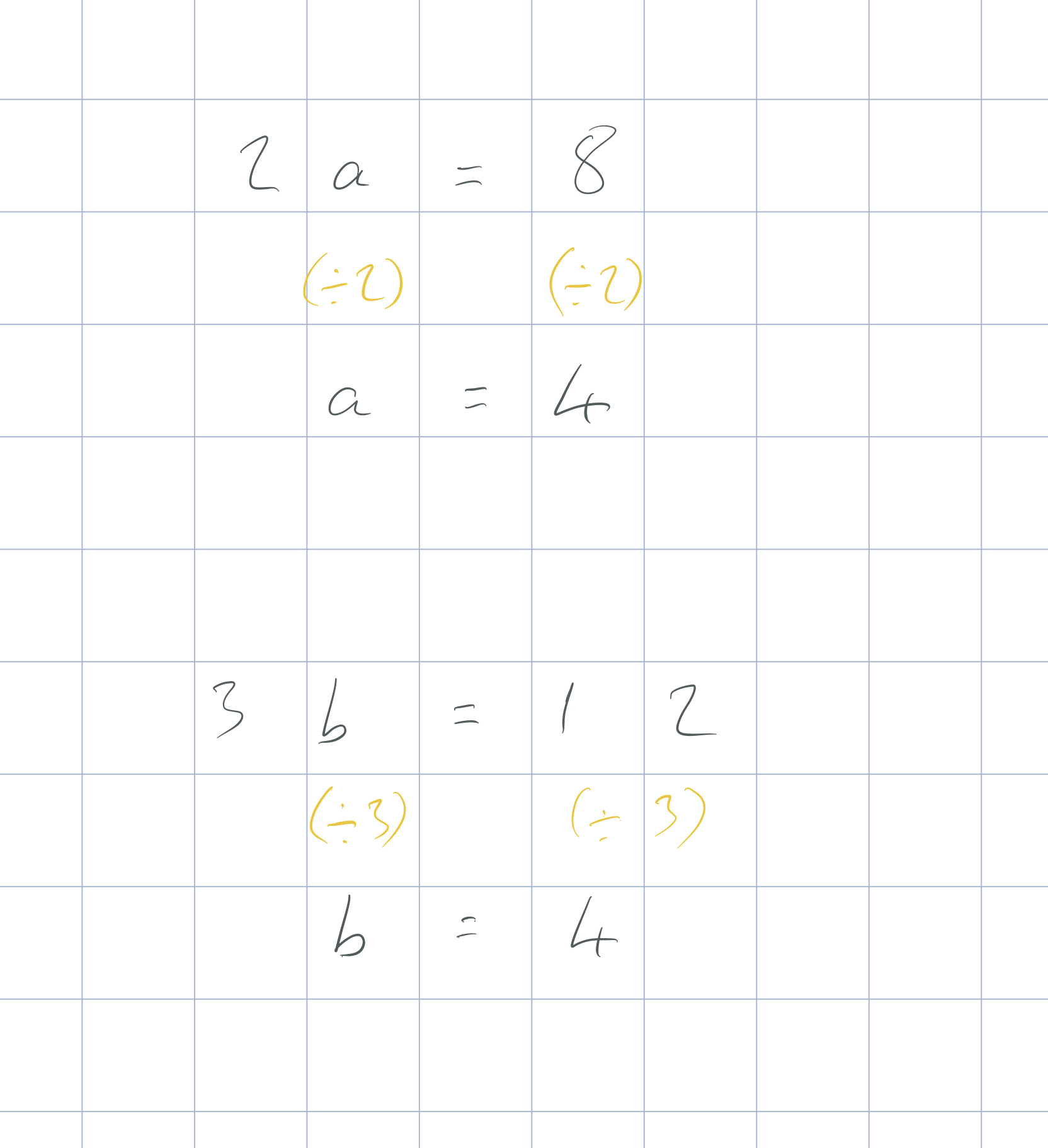To solve equations,  we undo all of the operations on the side of the equation with the letters. In the last exercise, we added or subtracted something to both sides of the equation, in this section, we divide both sides.

In the equation 2a = 8, 2a means 2 × a. The opposite of 2 × a is ÷ 2.